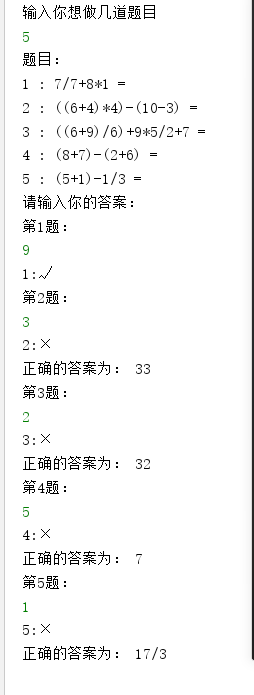## python_四则运算 转

o
osc_y8yehimr

### Github项目地址

https://github.com/LWX1/-

【小学生的四则运算】--PSP表格

 psp 任务计划 计划完成需要的时间（min） 实际完成需要的时间 Planning 计划 120 60 Estimate 估计这个任务需要多少时间，并 规划大致工作时间 70 60 Development 开发 1000 500 Analysis 需要分析（包括学习新技术） 100 30 Design Spec 生成设计文档 10 5 Design Review 设计复审 20 30 Coding Standard 代码规范 20 20 Design 具体设计 60 30 Coding 具体编码 300 400 Code Review 代码复审 100 30 Test 测试（自我测试，修改代码，提交代码） 10 20 Reporting 报告 60 40 Test Report 测试报告 60 120 Size Measurement 计算工作量 10 10 Postmortem & Process Improvement Plan 事后总结，并提出改进计划 60 30 合计 2000 1385

## 项目要求

1、能自动生成小学四则运算题目

2、题目中不能出现负数或者结果为负数

3、除了整数的运算还包括真分数的运算

1、自动产生括号

2、随机生成多个表达式

# 思路描述

1. 列出随机的四则运算表达式。
2. 计算所列出四则运算的结果。
3. 接受用户输入并比较结果是否正确，并在命令行中表现出来。

### 如何解决

#### 问题1

• 随机操作数、随机运算符、随机括号、随机长度等随机变量可以利用python自带随机函数取得。
• 需要考虑除数及分母为0时的情况，此时表达式不成立。

#### 问题2

• python中有一个函数eval能计算表达式的结果，但结果可能会为分数，要利用库fractons中的一个函数Fraction，把输出的结果全转成分数，所以整数的不变，小数的变分数
• 再使用一个result的列表存储每一个表达式结果，再用for提取其中的每个结果

#### 问题三

• 利用input（）函数输入答案，再用eval和Fraction转成数字。

#### 具体程序设计

 变量 类型 作用 question list 存储表达式 answer list 存储回答的答案 result list 存储表达式的结果答案 f str 把列表中的值合成表达式 operation list 存储运算符

 函数名 作用 ``integer（n）`` 随机产生几个表达式 ``func_integer(number)`` 表达式的随机生成与是否需要括号 ``result_integer(f, m)`` 根据表达式计算结果

#### #integer 函数`````` 1 def integer(n):
2     ch = []                               #存储表达式
3     number = random.randint(1, 4)        #随机产生表达式的数量
4     for i in range(number):
5         rand = random.randint(0, 1)       #随机产生0和1 判断是否使用括号
6         a = func_integer(number)          #调用表达式产生函数，产生表达式
7         if rand == 0:
8             op = operation[random.randint(2,3)]    #产生*，/来连接有括号的表达式，避免产生+，—
9             rand = random.randint(0, 1)            #随机产生0和1 判断是否使用内嵌括号或外嵌括号
10             if i != number - 1:                    #避免开始和结尾用无意义的括号
11                 if rand == 0:
12                     ch.append('(')
13                     ch.append(a)
14                     ch.append(op)
15                     ch.append(random.randint(1,10))
16                     ch.append(')')
17                     ch.append(operation[random.randint(0, 3)])
18                 else:
19                     ch.append(a)
20                     ch.append(operation[random.randint(0, 3)])
21             else:
22                 ch.append(a)
23                 ch.append(operation[random.randint(0, 3)])
24         else:
25             ch.append(a)
26             ch.append(operation[random.randint(0, 3)])
27
28     f = ''
29     for k,i in enumerate(ch):             #把列表中的所有值用f一个个连起来
30         if k != len(ch)-1:
31             f += str(i)
32     result_integer(f, n)                      #调用输出函数``````
View Code

#### #func_integer() 函数`````` 1 def func_integer(number):
2     f = ''
3     ch = []
4     rand = random.randint(0, 1)  #选择内嵌或外嵌括号
5     if number != 1:         #避免一个表达式也产生括号
6         if rand == 0:
7             ch.append('(')
8             op = operation[random.randint(0, 1)]
9             ch.append(random.randint(1, 10))
10             ch.append(op)
11             ch.append(random.randint(1, 10))
12             ch.append(')')
13         else:
14             op = operation[random.randint(0, 3)]
15             if op == '/':
16                 a = random.randint(1, 10)
17                 ch.append(a)
18                 ch.append(op)
19                 ch.append(random.randint(a, 10))
20             else:
21                 ch.append(random.randint(1, 10))
22                 ch.append(op)
23                 ch.append(random.randint(1, 10))
24     else:
25         op = operation[random.randint(0, 3)]
26         if op == '/':
27             a = random.randint(1, 10)
28             ch.append(a)
29             ch.append(op)
30             ch.append(random.randint(a, 10))
31         else:
32             ch.append(random.randint(1, 10))
33             ch.append(op)
34             ch.append(random.randint(1, 10))
35     for i in ch:       #把产生表达式当成一个整体
36         f += str(i)
37     return f``````
View Code

#### #result_integer（）函数`````` 1 def result_integer(f, m):
2     try:
3         n = eval(f)
4         n = Fraction('{}'.format(n)).limit_denominator()  # 把表达式的结果转成分数
5         if n > 0:  # 判断结果是否大于0，否则重新产生表达式
6             #print('题目：')
7             question.append(f)
8             result.append(n)
9             #print('请输出答案：')
10             #x = Fraction('{}'.format(eval(input()))).limit_denominator()
11         else:
12             integer()
13     except:
14         integer(m)``````
View Code

``代码运行如下``## 总结及收获

o

### osc_y8yehimr#### 暂无文章

Springboot+Redis综合运用/缓存使用

42分钟前
5
0

17
0

19
0
Java线程池

nullpointerxyz

57
0
Python笔记：用Python制作二维码

tengyulong

9
0